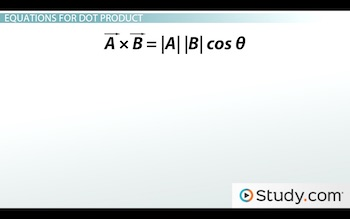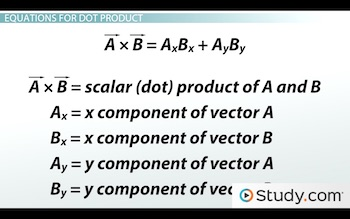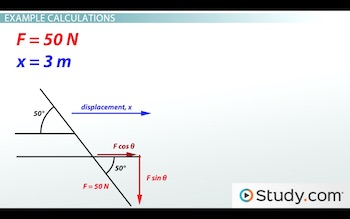# The Dot Product and Vectors: Definition & Formula

An error occurred trying to load this video.

Try refreshing the page, or contact customer support.

Coming up next: Finding The Cross Product of Two Vectors

### You're on a roll. Keep up the good work!

Replay
Your next lesson will play in 10 seconds
• 0:00 What is a Dot Product?
• 1:24 Equations for Dot Product
• 2:30 Example Calculations
• 4:20 Lesson Summary
Save Save

Want to watch this again later?

Timeline
Autoplay
Autoplay
Speed Speed Audio mode

#### Recommended Lessons and Courses for You

Lesson Transcript
Instructor
David Wood

David has taught Honors Physics, AP Physics, IB Physics and general science courses. He has a Masters in Education, and a Bachelors in Physics.

Expert Contributor
Matthew Bergstresser

Matthew has a Master of Arts degree in Physics Education. He has taught high school chemistry and physics for 14 years.

After watching this lesson, you will be able to explain what a dot product is, how it is different from a cross product, and use equations to calculate dot products. A short quiz will follow.

## What Is a Dot Product?

A vector is a quantity that has both magnitude (numerical size) AND direction. A scalar, on the other hand, is a quantity that has only magnitude - it's just a number. Vectors are things like velocity, displacement, force, or electric field. Those quantities always have a direction. A velocity isn't 3 m/s; it's 3 m/s NORTH. But a scalar would be something like temperature, time or distance. Those are defined by their numerical values - they're not pointed in any particular direction.

When we multiply two numbers together that happen to be vectors, we do it in different ways depending on the circumstances. If the result you'll be getting is a vector quantity - if it has a direction itself - then you have to do a cross product. But if the result you want is a scalar quantity, a quantity that doesn't have a direction, then you use a dot product. A dot product is where you multiply one vector by the component of the second vector, which acts in the direction of the first vector.

So, for example, work is force multiplied by displacement. It's two vectors multiplied together. But more specifically it's the force acting in the direction you're moving, multiplied by the displacement. This is why work is a dot product. Of course, the fact that work is a scalar quantity, not a vector, might also have tipped you off.

## Equations for Dot Product

There are two equations for dot products. One for when you have the overall magnitudes and angles of the vector, which looks like this:And another, if you're given the two vectors in component form, which looks like this:So, for the first, if you're multiplying vector A and vector B together, you take the magnitude of vector A, multiply it by the magnitude of vector B, and multiply that by the cosine of the angle between them.

But for the second equation, a situation where you don't know the overall magnitudes, you multiply the x components and y components together separately and then add them up. The answer works out exactly the same.

So, with our example of work, if you know that the force is 3 newtons and the displacement is 2 meters and the angle between the force and displacement is 30 degrees, you multiply 3 by 2 by cosine 30 and you get your answer. But, if instead you're told that the force is 30i + 8j newtons, and the displacement is 15i + 15j meters, you would instead use the second equation. It's all based on what information you're given.

## Example Calculations

Alright, let's go through an example. Imagine you're sweeping the floor with a broom. You're pushing down on the broom with a force of 50 newtons, and the broom is at a 50 degree angle with the floor. So you're pushing partly down and partly forwards. If you push the broom 3 meters across the floor, how much work is done?

Well, work is a scalar and is equal to force multiplied by displacement, so we definitely want to do a dot product (I'm sure you're very surprised). First of all, let's write out what we know. The force, F, is 50 newtons and the displacement, x, is 3 meters.Looking at this diagram, we can see that the angle between the force and the displacement is the same as the angle between the broom and the floor - 50 degrees. So theta equals 50 degrees. Plug all that into our dot product equation, and we get 50 multiplied by 3 multiplied by cosine 50, and that gives us 96.4 Joules of work. And that's it; that's our answer.

To unlock this lesson you must be a Study.com Member.

## The Dot Product and Vectors

The dot product is a method to multiply two vectors that results in a scalar. Calculating work in physics requires the dot product. Let's work through some problems utilizing the dot product. Solve the problems on your own without a look at the solutions. After you are done, check your answers under the solutions section.

### Practice Problems

1. Determine the dot product between vector a, which has a magnitude of 2 and vector b, which has a magnitude of 6. The angle between vectors a and b is 30°.

2. The dot product of two vectors is 3, vector p has a magnitude of 2, and vector q has a magnitude of 4. What is the angle between vectors p and q?

3. Vector a is 2 units east and 3 units north. Vector b is 4 units west and 2 units south. Determine the dot product of vectors a and b.

4. Determine the work done by a force of 10 N applied at a 90° angle to the displacement of the object, which is 20 m. Work is the dot product of the force on the object and displacement of the object.

### Solutions

1. abcosθ = (2)(6)cos30° = 10.4

2.

pqcosθ = the dot product

(2)(4)cosθ = 3

8cosθ = 3

cosθ = 3/8

θ = 68°

3. ax bx + ay by = (2)(3) + (-4)(-2) = 6 + 8 = 14

4.

W = Fdcosθ

W = (10)(20)cos90°

W = 0 joules

Work can only be done on an object if a component or all of the force exerted on it is parallel or antiparallel to the object's displacement.

### Register to view this lesson

Are you a student or a teacher?

#### See for yourself why 30 million people use Study.com

##### Become a Study.com member and start learning now.
Back
What teachers are saying about Study.com

### Earning College Credit

Did you know… We have over 200 college courses that prepare you to earn credit by exam that is accepted by over 1,500 colleges and universities. You can test out of the first two years of college and save thousands off your degree. Anyone can earn credit-by-exam regardless of age or education level.